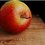# Exploring the Divisor Function

This note is part of the set Exploring the Divisor Function.

In this set, we aim to get a general form for this sum:

$\sum _{n=1}^{\infty}\frac{d\left(kn\right)}{n^2}$

Where $k$ is a positive integer.

So, instead of giving out everything on a note, why not split it up into several problems so that everybody can try it out by themselves?

I will give a clue here, and then you can go ahead to solve the first problem of this set, slowly progressing to the last problem, where you will finally be able to find a general form of the sum. You may skip steps, because your approach might be better than mine. If you do have a better approach, do post it!

Here's the first clue:

If $f(n)$ is completely multiplicative, that is $f(ab)=f(a)f(b)$, then

$f*f(n)=d(n)f(n)$

$\left[\sum _{n=1}^{ \infty}\frac{f\left(n\right)}{n^s}\right]^2=\sum _{n=1}^{\infty }\frac{f\left(n\right)d\left(n\right)}{n^s}$

Where $*$ is the Dirichlet Convolution

and $d(n)$ counts the number of divisors n.

I would post the solutions for the problems soon.Note by Julian Poon
5 years, 4 months ago

This discussion board is a place to discuss our Daily Challenges and the math and science related to those challenges. Explanations are more than just a solution — they should explain the steps and thinking strategies that you used to obtain the solution. Comments should further the discussion of math and science.

When posting on Brilliant:

• Use the emojis to react to an explanation, whether you're congratulating a job well done , or just really confused .
• Ask specific questions about the challenge or the steps in somebody's explanation. Well-posed questions can add a lot to the discussion, but posting "I don't understand!" doesn't help anyone.
• Try to contribute something new to the discussion, whether it is an extension, generalization or other idea related to the challenge.
• Stay on topic — we're all here to learn more about math and science, not to hear about your favorite get-rich-quick scheme or current world events.

MarkdownAppears as
*italics* or _italics_ italics
**bold** or __bold__ bold
- bulleted- list
• bulleted
• list
1. numbered2. list
1. numbered
2. list
Note: you must add a full line of space before and after lists for them to show up correctly
paragraph 1paragraph 2

paragraph 1

paragraph 2

[example link](https://brilliant.org)example link
> This is a quote
This is a quote
    # I indented these lines
# 4 spaces, and now they show
# up as a code block.

print "hello world"
# I indented these lines
# 4 spaces, and now they show
# up as a code block.

print "hello world"
MathAppears as
Remember to wrap math in $$ ... $$ or $ ... $ to ensure proper formatting.
2 \times 3 $2 \times 3$
2^{34} $2^{34}$
a_{i-1} $a_{i-1}$
\frac{2}{3} $\frac{2}{3}$
\sqrt{2} $\sqrt{2}$
\sum_{i=1}^3 $\sum_{i=1}^3$
\sin \theta $\sin \theta$
\boxed{123} $\boxed{123}$

## Comments

Sort by:

Top Newest

my mind is blown

- 5 years, 3 months ago

Log in to reply

I cannot work it out for 4 as $k$ Can you help?

- 5 years, 3 months ago

Log in to reply

Oh yeah, thanks for commenting here. I forgot to add the solutions...

If you want to consider k=4, generalise it to $k=p^a$, where p is prime. I'll post a solution to part 3 of the set by tomorrow, where you can use it for $k=4$.

You can use part 1 of this set as a clue to part 3, but you'll have to be more creative.

- 5 years, 3 months ago

Log in to reply

thanks!

- 5 years, 3 months ago

Log in to reply

I have posted a solution to part 3.

- 5 years, 2 months ago

Log in to reply

×

Problem Loading...

Note Loading...

Set Loading...